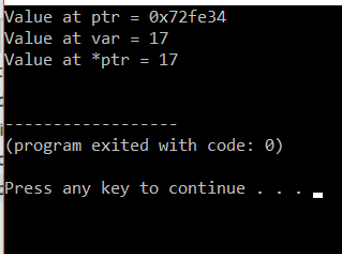AWS Global Infrastructure

### Programming & Frameworks

Topics Covered
• C Programming and Data Structures (16 Blogs)
• Comprehensive Java Course (4 Blogs)
• Java/J2EE and SOA (346 Blogs)
• Spring Framework (8 Blogs)
SEE MORE

# How To Implement Pointers In C++?How To Implement Pointers In C++?

Pointers in C++ is a variable that holds the address of another variable in c++. The address is stored in the pointer variable and help in the implementation of call-by-reference.

Syntax:

```
datatype *pointername;
EXAMPLE:
int *ptr;

```
• A pointer variable has a * sign before its name.
• A pointer is also called a locator or Indicator.

Uses Of Pointer:

• Dynamic Memory Allocation
• In Various Programs Of Arrays, Structure, And Functions

Here Is An Example Code:

```#include <iostream>
using namespace std;
int main()
{
int num = 17;
int *ptr;
ptr = &num;
cout << "Value at ptr = " << ptr << "n";
cout << "Value at var = " << num << "n";
cout << "Value at *ptr = " << *ptr << "n";
}
```

Output:Explanation:

In the above program, we show the basic working of a pointer. We have an integer variable num with value 17. We have pointer variable ptr of type int. We assign the address of num to the pointer ptr.

We first print the value of ptr, that is the address. Next, we print the num value and at the end, we print the value at the location held by the pointer ptr.

## Pointers and Arrays:

We can consider the first element of an array as a pointer, as the array name contains the address of the first element. We can use a pointer in the following fashion.

Here is an example code:

```
#include <iostream>
using namespace std;
int main()
{
int arr = { 5, 10, 20 };
int *ptr;
ptr = arr ;
cout << "Elements of the array are: ";
cout << ptr << " " << ptr << " " << ptr;
}

```
`Output:`Explanation:

In the above program, we show the basic working of a pointer with an array. We have an array arr with values 5,10,20. We have pointer variable ptr of type int. We assign the address of arr to the pointer ptr.

We first print the value of ptr, that is the first element of the array. Next, we print the second and third elements respectively. As array elements are stored consecutively, so the pointer can access the other location of the array by incrementation.

## Null Pointers:

There are the type of pointers that have no value and hold a null value

Example:

`int *ptr=NULL;`

They are very useful in data structures like a linked list.

## Void Pointers:

These are the type of pointers that do not have a return type.

## Pointer Arithmetic Operation:

Different operations can be performed on pointers. Here are some important types.

• incremented (++)
• decremented ( — )
• difference between two pointers (p1-p2)
• addition of an integer to a pointer ( + or += )
• subtraction of an integer from a pointer ( – or -= )

Here is a code to demo some of these operations:

```#include <iostream>
using namespace std;
int main()
{
int arr = {10, 100, 200};
int *ptr;
ptr = arr;
for (int i = 0; i < 3; i++)
{
cout << "Value at different locations of array using *ptr = " << *ptr << "n";
ptr++;
}
}
```

Output:Explanation:

We demonstrate simple arithmetic operation of incrementation of pointer variable is shown in the above program.

## Pointer to Pointer:

In this type of system, there are two pointers. The first pointer points to the second pointer and the second pointer points to the variable that is holding the value.

Here is an Example Code:

```</pre>
#include <iostream>
using namespace std;
int main () {
int num;
int *ptr;
int **pptr;
num = 3000;
ptr = &num;
pptr = &ptr;
cout << "Value of num :" << num<< endl;
cout << "Value available at *ptr :" << *ptr << endl;
cout << "Value available at **pptr :" << **pptr << endl;
return 0;
}

```

Output:## Pointer to Functions:

This is a way of passing pointers to functions. The function parameter must be declared as a pointer type. It is shown in the code below,

```#include <iostream>
using namespace std;
float getAverage(int *arr, int size);
int main ()
{
int balance = {1432, 232, 3232, 17, 502};
float avg;
avg = getAverage( balance, 5 ) ;
cout << "Average value is: " << avg << endl;
return 0;
}
float getAverage(int *arr, int size) {
int i, sum = 0;
double avg;
for (i = 0; i < size; ++i) {
sum += arr[i];
}
avg = double(sum) / size;
return avg;
}
```

OutputThis is how we pass a pointer to a function.

Thus we have come to an end of this article on ‘Pointers in C++’. If you wish to learn more, check out the Java Training by Edureka, a trusted online learning company. Edureka’s Java J2EE and SOA training and certification course is designed to train you for both core and advanced Java concepts along with various Java frameworks like Hibernate & Spring.

Got a question for us? Please mention it in the comments section of this blog  and we will get back to you as soon as possible.REGISTER FOR FREE WEBINARThank you for registering Join Edureka Meetup community for 100+ Free Webinars each month